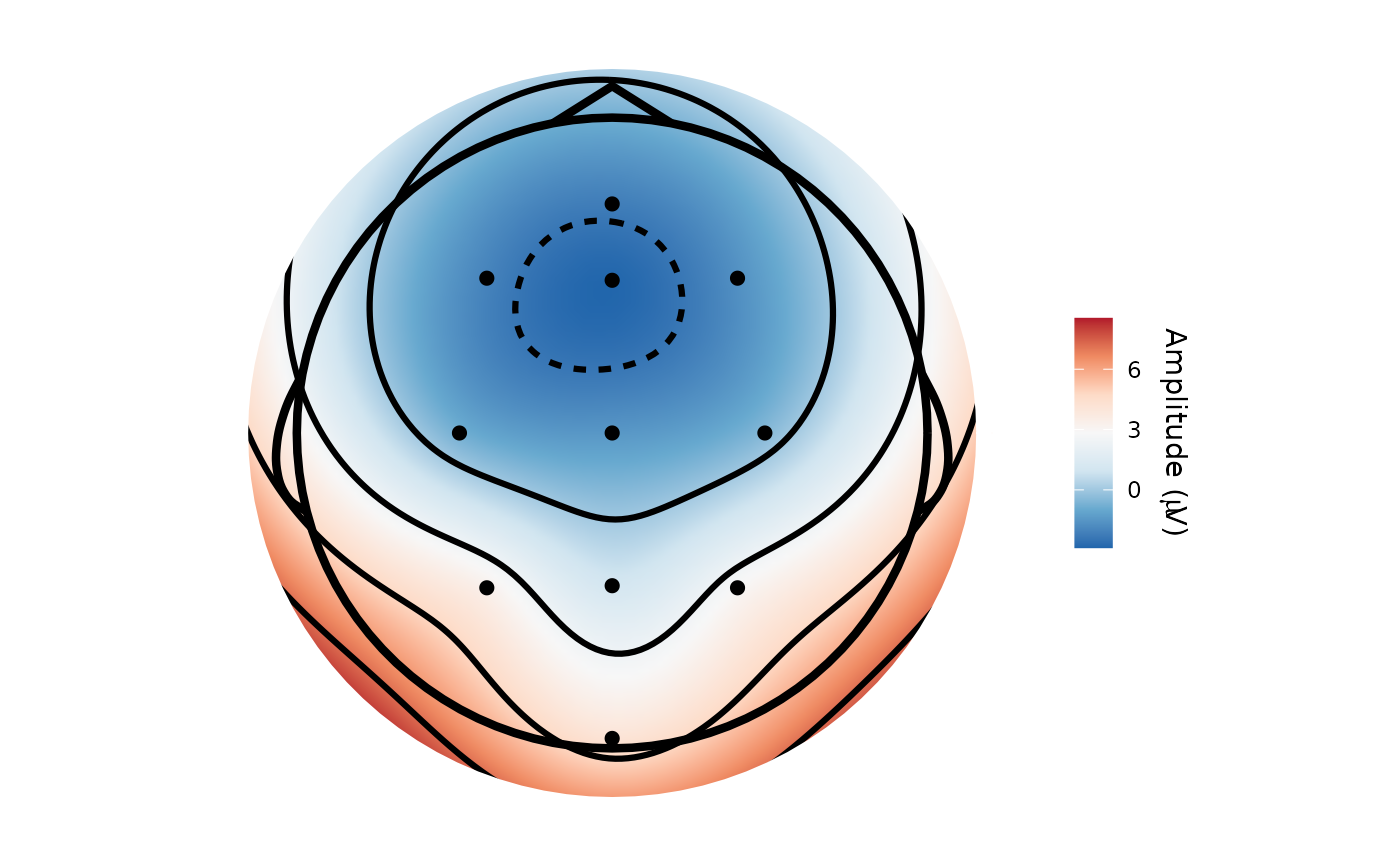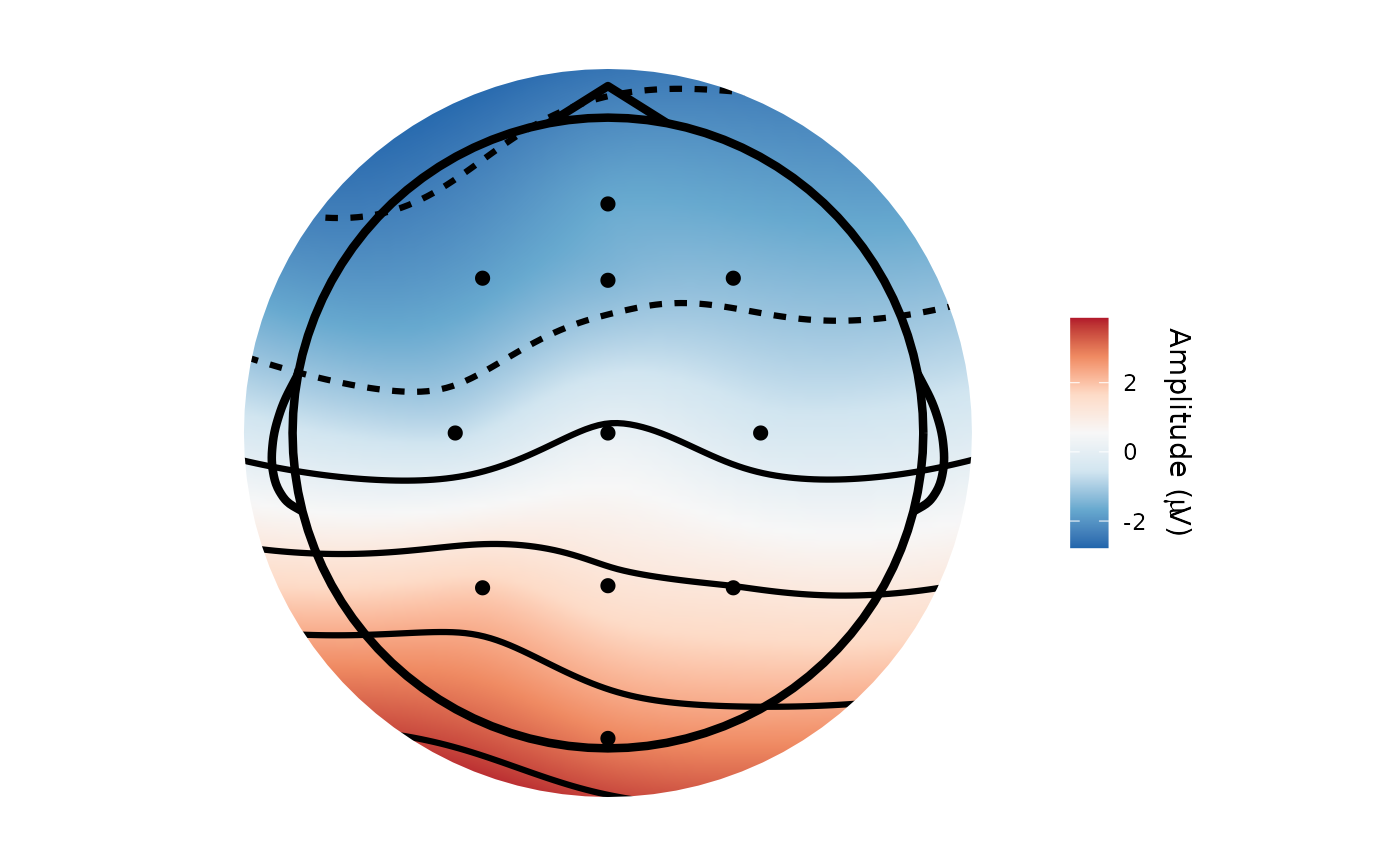Allows topographical plotting of functional data. Output is a ggplot2 object.

topoplot(data, ...)

# S3 method for default
topoplot(data, ...)

# S3 method for data.frame
topoplot(
data,
time_lim = NULL,
limits = NULL,
chanLocs = NULL,
method = "Biharmonic",
r = NULL,
grid_res = 200,
palette = "RdBu",
interp_limit = "skirt",
contour = TRUE,
chan_marker = "point",
quantity = "amplitude",
montage = NULL,
highlights = NULL,
scaling = 1,
groups = NULL,
verbose = TRUE,
k = 40,
...
)

# S3 method for eeg_data
topoplot(
data,
time_lim = NULL,
limits = NULL,
chanLocs = NULL,
method = "Biharmonic",
r = NULL,
grid_res = 200,
palette = "RdBu",
interp_limit = "skirt",
contour = TRUE,
chan_marker = "point",
quantity = "amplitude",
montage = NULL,
highlights = NULL,
scaling = 1,
verbose = TRUE,
groups = NULL,
k = 40,
...
)

# S3 method for eeg_epochs
topoplot(
data,
time_lim = NULL,
limits = NULL,
chanLocs = NULL,
method = "Biharmonic",
r = NULL,
grid_res = 200,
palette = "RdBu",
interp_limit = "skirt",
contour = TRUE,
chan_marker = "point",
quantity = "amplitude",
montage = NULL,
highlights = NULL,
scaling = 1,
groups = NULL,
verbose = TRUE,
k = 40,
...
)

# S3 method for eeg_ICA
topoplot(
data,
component,
time_lim = NULL,
limits = NULL,
chanLocs = NULL,
method = "Biharmonic",
r = NULL,
grid_res = 200,
palette = "RdBu",
interp_limit = "skirt",
contour = TRUE,
chan_marker = "point",
quantity = "amplitude",
montage = NULL,
highlights = NULL,
scaling = 1,
verbose = TRUE,
groups = NULL,
k = 40,
...
)

# S3 method for eeg_tfr
topoplot(
data,
time_lim = NULL,
limits = NULL,
chanLocs = NULL,
method = "Biharmonic",
r = NULL,
grid_res = 200,
palette = "RdBu",
interp_limit = "skirt",
contour = TRUE,
chan_marker = "point",
quantity = "power",
montage = NULL,
highlights = NULL,
scaling = 1,
freq_range = NULL,
verbose = TRUE,
groups = NULL,
k = 40,
...
)

## Arguments

data

An EEG dataset. If the input is a data.frame, then it must have columns x, y, and amplitude at present. x and y are (Cartesian) electrode co-ordinates), amplitude is amplitude.

...

Various arguments passed to specific functions

time_lim

Timepoint(s) to plot. Can be one time or a range to average over. If none is supplied, the function will average across all timepoints in the supplied data.

limits

Limits of the fill scale - should be given as a character vector with two values specifying the start and endpoints e.g. limits = c(-2,-2). Will ignore anything else. Defaults to the range of the data.

chanLocs

Allows passing of channel locations (see electrode_locations)

method

Interpolation method. "Biharmonic" or "gam". "Biharmonic" implements the same method used in Matlab's EEGLAB. "gam" fits a Generalized Additive Model with k = 40 knots. Defaults to biharmonic spline interpolation.

r

Radius of cartoon head_shape in mm. Default value is 95 (mm) when using interp_limit = "skirt".

grid_res

Resolution of the interpolated grid. Higher = smoother but slower. Defaults to 200 points per edge.

palette

Defaults to RdBu if none supplied. Can be any palette from RColorBrewer or viridis. If an unsupported palette is specified, switches to Greens.

interp_limit

"skirt" or "head". Defaults to "skirt". "skirt" interpolates just past the farthest electrode and does not respect the boundary of the head_shape. "head" interpolates up to the radius of the plotted head, and moves all electrodes inside the head.

contour

Plot contour lines on topography (defaults to TRUE)

chan_marker

Set marker for electrode locations. "point" = point, "name" = electrode name, "none" = no marker. Defaults to "point".

quantity

Allows plotting of an arbitrary quantitative column. Defaults to amplitude. Use quoted column names. E.g. "p.value", "t_statistic".

montage

Name of an existing montage set. Defaults to NULL; (currently only 'biosemi64alpha' available other than default 10/20 system)

highlights

Electrodes to highlight (in white).

scaling

Scaling multiplication factor for labels and any plot lines. Defaults to 1.

groups

Column name for groups to retain. This is required to create facetted plots.

verbose

Warning messages when electrodes do not have locations. Defaults to TRUE.

k

Degrees of freedom used for spline when using method = gam. Defaults to 40.

component

Component to plot (numeric)

freq_range

Range of frequencies to average over.

## Methods (by class)

• default: Default method for data frames.

• data.frame: Topographical plotting of data.frames and other non eeg_data objects.

• eeg_data: Topographical plotting of eeg_data objects.

• eeg_epochs: Topographical plotting of eeg_epochs objects.

• eeg_ICA: Topographical plot for eeg_ICA objects

• eeg_tfr: Topographical plotting of eeg_tfr objects.

## Notes on usage of Generalized Additive Models for interpolation

The function fits a GAM using the gam function from mgcv. Specifically, it fits a spline using the model function gam(z ~ s(x, y, bs = "ts", k = 40). Using GAMs for smooths is very much experimental. The surface is produced from the predictions of the GAM model fitted to the supplied data. Values at each electrode do not necessarily match actual values in the data: high-frequency variation will tend to be smoothed out. Thus, the method should be used with caution. In addition the method can only be used when there are more than 40 electrodes.

Other scalp-based maps: erp_scalp(), interactive_scalp()

## Author

topoplot(demo_epochs)